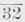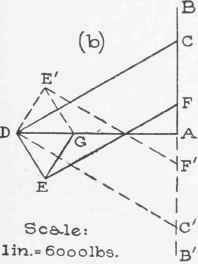Memberbeing in tension, pulls down on joint (3) as shown in Fig. 20 (d). The other forces acting on that joint are the load 1,800 pounds, the push 1,875 pounds, the pull 600 pounds, and the force exerted by memberwhich we will call F5. The only one of these forces having horizontal components are 1,875 and F5; hence in order that these two components may balance, F5 must act toward the left. F5 is therefore a push and the memberis under compression.

* The angles can be computed from the dimensions of the truss; often they can be ascertained easiest by scaling them with a protractor from a large size drawing of the truss.

The horizontal component of 1,875 = 1,875 X cos 53° 8' = 1,125; and the horizontal component of F5 = F5 X cos 38° 40' = 0.7808 F5.

Hence 0.7808 F5 = 1,125, or, F5 =1,125/.7808=1,440 pounds.

(This same truss is analyzed graphically later.)

24. Notation for Graphical Analysis of Trusses. The nota. tion described in Art. 3 can be advantageously systematized in this connection as follows: Each triangular space in the diagram of the truss and the spaces between consecutive lines of action of the loads and reactions should be marked by a small letter (seeFig. 21.

Fig. 21 a). Then the two letters on opposite sides of any line serve to denote that line and the same large letters are used to denote the force acting in that line. Thus cd (Fig. 21 a) refers to the memberand CD should be used to stand for the force or stress in that member.

25. Polygon for a Joint. In drawing the polygon for all the forces at a joint, it is advantageous to represent the forces in the order in which they occur about the joint. Evidently there are always two possible orders thus (see Fig. 20 d) F5, 600, 1,875, and 1,800 is one order around, and F5, 1,800, 1,875, and GOO is another. The first is called a clockwise order and the second counter-clockwise.

A force polygon for the forces at a joint in which the forces are represented in either order in which they occur about the joint is called a polygon for the joint, and it will be called a clockwise or counter-clockwise polygon according as the order followed is clockwise or counter-clockwise. Thus in Fig. 17 (b), ABCDA is a clockwise polygon for joint (1). ABCD'A is a polygon for the forces at the joint; it is not a polygon for the joint because the order in which the forces are represented in that polygon is not the same as either order in which they occur about the joint.Fig. 22.

(Draw the counter-clockwise polygon for the joint and compare it with ABCDA and ABCD'A.)

26. Stress Diagrams. If the polygons for all the joints of a truss are drawn separately as in Example 1, Art. 23, the stress in each member will have been represented twice. It is possible to combine the polygons so that it will be unnecessary to represent the stress in any one member more than once, thus reducing the number of lines to be drawn. Such a combination of force polygons is called a stress diagram.

Fig 21 (5) is a stress diagram for the truss of Fig. 21 (a) same as the truss of Fig. 16. It will be seen that the part of the stress diagram consisting of solid lines is a combination of separate polygons previously drawn for the joints on the left half of the truss (Figs. 17, 18 and 19.) It will also be seen that the polygons are all clockwise, but counter-clockwise polygons could be combined into a stress diagram.

To Construct a Stress Diagram for a Truss Under Given Loads.

1. Determine the reactions*.

2. Letter the truss diagram as explained in Art. 24.

3. Construct a force polygon for all the forces applied to the truss (loads and reactions) representing them in the order in which they occur around the truss, clockwise or counter-clockwise. (The part of this polygon representing the loads is called a load line.)

4. On the sides of that polygon, construct the polygons for all the joints. They must be clockwise or counter-clockwise according as the polygon for the loads and reactions is clockwise or counter-clockwise. (The first polygon for a joint must be drawn for one at which but two members are connected - the joints at the supports are usually such. Then one can draw in succession the polygons for joints at which there are not more than two unknown forces until the stress diagram is completed.)

Example. It is desired to construct a stress diagram for the truss represented in Fig. 22 (a), it being supported at its ends and sustaining two loads of 1,800 and 600 pounds as shown.

The right and left reactions are 900 and 1,500 pounds as is shown in Example 1, Art. 37. Following the foregoing directions we first letter the truss, as shown. Then, where convenient, draw the polygon for all the loads and reactions, beginning with any force, but representing them in order as previously directed. Thus, beginning with the 1,800-pound load and following the clockwise order for example, lay off a line 1.8 inch in length representing 1,800 pounds (scale 1,000 pounds to an inch); since the line of action of the force is bc, the line is to be marked BC and B should be placed at the upper end of the line for a reason which will presently appear. The next force to be represented is the right reaction, 900 pounds ; hence from C draw a line upward and 0.90 inch long;. The line of action of this force being ce, the line just drawn should be marked CE and since C is already at the lower end, we mark the upper end E. (The reason for placing B at the upper end of the first line is now apparent.) The next force to be represented is the 600-pound load ; therefore we draw from E a line downward and 0.6 inch long, and since the line of action of that force is ef, mark the lower end of the line F. The next force to be represented is the left reaction, 1,500 pounds, hence we draw a line 1.5 inches long and upward from F. If the lines have been carefully laid off, the end of the last line should fall at B, that is, the polygon should close.